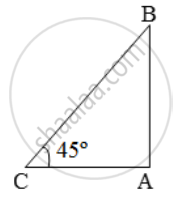# A ladder against a vertical wall makes an angle of 45º with the ground. The foot of the ladder is 3m from the wall. Find the length of the ladder - Mathematics

Sum

A ladder against a vertical wall makes an angle of 45º with the ground. The foot of the ladder is 3m from the wall. Find the length of the ladder

#### Solution

Let AB be the wall and CB, the ladder.Then, AC = 3m and ∠ACB = 45º

\text{Now, }\frac{CB}{AC}=\sec \text{45}^\text{o}=\sqrt{2}\Rightarrow \frac{CB}{3}=\sqrt{2}

∴ Length of the ladder = CB = 3 √2

= (3 × 1.41) m = 4.23 m

Concept: Heights and Distances
Is there an error in this question or solution?